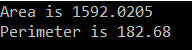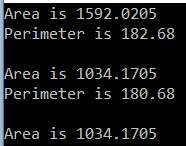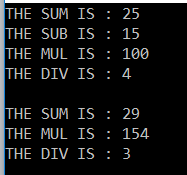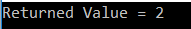# Multicast Delegates in C#

## Multicast Delegates in C# with Examples

In this article, I am going to discuss the Multicast Delegates in C# with examples. Please read our previous article before proceeding to this article where we discussed Single Cast Delegates in C# with examples. As part of this article, we are going to discuss the following pointers in detail.

1. What is Multicast Delegate in C#?
2. How to create Multicast Delegates in C#?
3. Different ways to create Multicast Delegates.
4. Multicast Delegate with Return Type in C#.
5. Multicast Delegate with Output Parameter in C#.
##### What is Multicast Delegate in C#?

A Multicast Delegate is a delegate that holds the references of more than one function. When we invoke the multicast delegate, then all the functions which are referenced by the delegate are going to be invoked. If you want to call multiple methods using a delegate then all the method signature should be the same.

##### Let us see an example for understanding the Multicast Delegate.
```namespace MulticastDelegateDemo
{
public class Rectangle
{
public void GetArea(double Width, double Height)
{
Console.WriteLine(@"Area is {0}", (Width * Height));
}
public void GetPerimeter(double Width, double Height)
{
Console.WriteLine(@"Perimeter is {0}", (2 * (Width + Height)));
}
static void Main(string[] args)
{
Rectangle rect = new Rectangle();
rect.GetArea(23.45, 67.89);
rect.GetPerimeter(23.45, 67.89);
}
}
}```

OUTPUT:In the above example, we created an instance of the Rectangle class and then called the two methods. Now I want to create a single delegate that is going to invoke the above two methods (i.e. GetArea and GetPerimeter). The two methods having the same signature so we can create a single delegate that holds the reference of the above two methods. So, when we invoke the delegate, it is going to invoke the above two methods. And when we do so, then it is called a Multicast Delegate.

##### Let’s see how to create a Multicast Delegate in C#.
```namespace MulticastDelegateDemo
{
public delegate void RectangleDelete(double Width, double Height);
public class Rectangle
{
public void GetArea(double Width, double Height)
{
Console.WriteLine(@"Area is {0}", (Width * Height));
}
public void GetPerimeter(double Width, double Height)
{
Console.WriteLine(@"Perimeter is {0}", (2 * (Width + Height)));
}
static void Main(string[] args)
{
Rectangle rect = new Rectangle();
RectangleDelete rectDelegate = new RectangleDelete(rect.GetArea);
//RectangleDelete rectDelegate = rect.GetArea;

//binding a method with delegate object
// In this example rectDelegate is a multicast delegate. You use += operator
// to chain delegates together and -= operator to remove.

rectDelegate += rect.GetPerimeter;
rectDelegate(23.45, 67.89);

Console.WriteLine();
rectDelegate.Invoke(13.45, 76.89);

Console.WriteLine();
//Removing a method from delegate object
rectDelegate -= rect.GetPerimeter;
rectDelegate.Invoke(13.45, 76.89);

}
}
}```

OUTPUT:##### Another approach to Create Multicast Delegates in C#.

Here in the below example, I am going to show you the use both static and non-static methods along with different ways to create and invoke delegates.

```namespace MulticastDelegateDemo
{
public delegate void MathDelegate(int No1, int No2);

public class Program
{
public static void Add(int x, int y)
{
Console.WriteLine("THE SUM IS : " + (x + y));
}
public static void Sub(int x, int y)
{
Console.WriteLine("THE SUB IS : " + (x - y));
}
public void Mul(int x, int y)
{
Console.WriteLine("THE MUL IS : " + (x * y));
}
public void Div(int x, int y)
{
Console.WriteLine("THE DIV IS : " + (x / y));
}

static void Main(string[] args)
{
Program p = new Program();
MathDelegate del2 = new MathDelegate(Program.Sub);
MathDelegate del3 = p.Mul;
MathDelegate del4 = new MathDelegate(p.Div); ;

//In this example del5 is a multicast delegate. We can use +(plus)
// operator to chain delegates together and -(minus) operator to remove.
MathDelegate del5 = del1 + del2 + del3 + del4;
del5.Invoke(20, 5);
Console.WriteLine();
del5 -= del2;
del5(22, 7);

}
}
}```
###### OUTPUT:##### Multicast Delegates with Return Type in C#:

A multicast delegate invokes the methods in the invocation list, in the same order in which they are added.

If the delegate has a return type other than void and if the delegate is a multicast delegate. then only the value of the last invoked method will be returned. Along the same lines, if the delegate has an out parameter, the value of the output parameter will be the value assigned by the last method.

Let’s understand this concept with an example.

```namespace MulticastDelegateDemo
{
// Deletegate's return type is int
public delegate int SampleDelegate();
public class Program
{
static void Main()
{
SampleDelegate del = new SampleDelegate(MethodOne);
del += MethodTwo;

// The ValueReturnedByDelegate will be 2, returned by the MethodTwo(),
// as it is the last method in the invocation list.
int ValueReturnedByDelegate = del();
Console.WriteLine("Returned Value = {0}", ValueReturnedByDelegate);

}
// This method returns one
public static int MethodOne()
{
return 1;
}

// This method returns two
public static int MethodTwo()
{
return 2;
}
}
}```
###### OUTPUT:##### Multicast Delegates with output parameter in C#.
```namespace MulticastDelegateDemo
{
// Deletegate has an int output parameter
public delegate void SampleDelegate(out int Integer);

public class Program
{
static void Main()
{
SampleDelegate del = new SampleDelegate(MethodOne);
del += MethodTwo;

// The ValueFromOutPutParameter will be 2, initialized by MethodTwo(),
// as it is the last method in the invocation list.
int ValueFromOutPutParameter = -1;
del(out ValueFromOutPutParameter);

Console.WriteLine("Returned Value = {0}", ValueFromOutPutParameter);
}

// This method sets ouput parameter Number to 1
public static void MethodOne(out int Number)
{
Number = 1;
}

// This method sets ouput parameter Number to 2
public static void MethodTwo(out int Number)
{
Number = 2;
}
}
}```
###### OUTPUT:That’s it for today. In the next article, I am going to discuss one real-time example of using delegates. Here, In this article, I try to explain Multicast Delegates in C# with some examples. I hope you enjoy this article and understand the need and use of Multicast Delegates.

### 2 thoughts on “Multicast Delegates in C#”

1.Nice article, thanks!

2.In del multicasting what if one method is retuning void and one is returning some value ? E.g. del = new del(methodReturnInt); del += methodReturnVoid; del();